# NCERT Solutions For Class 6 Maths Chapter 5 Understanding Elementary Shapes

NCERT Solutions For Class 6 Maths Chapter 5 Understanding Elementary Shapes

### NCERT Solutions For Class 6 Maths Chapter 5 Understanding Elementary Shapes Ex 5.1

Free download NCERT Solutions for Class 6 Maths Chapter 5 Exercise 5.1, Ex 5.2, Ex 5.3, Ex 5.4, Ex 5.5, Ex 5.6 and Ex 5.7, 5.8 and 5.9 Understanding Elementary Shapes PDF for CBSE 2020 Exams.

### Understanding Elementary Shapes Class 6 Ex 5.1

Ex 5.1 Class 6 Maths Question 1.
What is the disadvantage in comparing line segment by metre observation?
Solution:
Comparing the lengths of two line segments simply by ‘observation’ may not be accurate. So we use divider to compare the length of the given line segments.

Ex 5.1 Class 6 Maths Question 2.
Why is it better to use a divider than a ruler, while measuring the length of a line segment?
Solution:
Measuring the length of a line segment using a ruler, we may have the following errors:
(i) Thickness of the ruler
(ii) Angular viewing
These errors can be eradicated by using the divider. So, it is better to use a divider than a ruler, while measuring the length of a line segment.

Ex 5.1 Class 6 Maths Question 3.[Note: If A, B, C are any three points on a line such AC + CB = AB, then we can be sure that C lies between A and B]
Solution:
Let us consider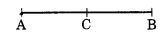A, B and C such that C lies between A and B and AB = 7 cm.
AC = 3 cm, CB = 4 cm.
∴ AC + CB = 3 cm + 4 cm = 7 cm.
But, AB = 7 cm.
So, AB = AC + CB.

Ex 5.1 Class 6 Maths Question 4.
If A, B, C are three points on a line such that AB = 5 cm, BC = 3 cm and AC = 8 cm, which one of them lies between the other two?
Solution:
We have, AB = 5 cm; BC = 3 cm
∴ AB + BC = 5 + 3 = 8 cm
But, AC = 8 cm
Hence, B lies between A and C.

Ex 5.1 Class 6 Maths Question 5.Solution:
From the given figure, we have
AG = 7 cm – 1 cm = 6 cm
AD = 4 cm – 1 cm = 3 cm
and DG = 7 cm – 4 cm = 3 cm
∴ AG = AD + DG.Ex 5.1 Class 6 Maths Question 6.Ex 5.1 Class 6 Maths Question 7.
Draw five triangles and measure their sides. Check in each case, if the sum of the length of any two sides is always less than the third side.
Solution:
Case I. In ∆ABCLet AB = 2.5 cm
BC = 4.8 cm
and AC = 5.2 cm
AB + BC = 2.5 cm + 4.8 cm
= 7.3 cm
Since, 7.3 > 5.2
So, AB + BC > AC
Hence, sum of any two sides of a triangle is greater than the third side.

Case II. In ∆PQR,Let PQ = 2 cm
QR = 2.5 cm
and PR = 3.5 cm
PQ + QR = 2 cm + 2.5 cm = 4.5 cm
Since, 4.5 > 3.5
So, PQ + QR > PR
Hence, sum of any two sides of a triangle is greater than the third side.

Case III. In ∆XYZ,Let XY = 5 cm
YZ = 3 cm
and ZX = 6.8 cm
XY + YZ = 5 cm + 3 cm
= 8 cm
Since, 8 > 6.8
So, XY + YZ > ZX
Hence, the sum of any two sides of a triangle is greater than the third side.

Case IV. In ∆MNS,Let MN = 2.7 cm
NS = 4 cm
MS = 4.7 cm
and MN + NS = 2.7 cm + 4 cm = 6.7 cm
Since, 6.7 >4.7
So, MN + NS > MS
Hence, the sum of any two sides of a triangle is greater than the third side.

Case V. In ∆KLM,Let KL = 3.5 cm
LM = 3.5 cm
KM = 3.5 cm
and KL + LM = 3.5 cm + 3.5 cm = 7 cm
7 cm > 3.5 cm
Solution:
(i) For one-fourth revolution, we have
So, KL + LM > KM
Hence, the sum of any two sides of a triangle is greater than the third side.
Hence, we conclude that the sum of any two sides of a triangle is never less than the third side.

### Understanding Elementary Shapes Class 6 Ex 5.2

Ex 5.2 Class 6 Maths Question 1.
What fraction of a clockwise revolution does the hour hand of a clock turn through, when it goes from
(a) 3 to 9
(b) 4 to 7
(c) 7 to 10
(d) 12 to 9
(e) 1 to 10
(f) 6 to 3
Solution:
(a) 3 to 9
9 – 3 = 6 ÷ 12 = 1/2 of a revolution

(b) 4 to 7
7 – 4 = 3 ÷ 12 = 1/4 of a revolution

(c) 7 to 10
10 – 7 = 3 ÷ 12 = 1/4 of a revolution

(d) 12 to 9 i.e., 0 to 9
9 – 0 = 9 ÷ 12 = 3/4 of a revolution

(e) 1 to 10
10 – 1 = 9 ÷ 12 = 3/4 of a revolution

(f) 6 to 3 i.e., 6 to 12 and then 12 to 3
6 to 12 = 12 – 6 = 6 and 12 to 3 = 0 to 3 = 3 – 0 = 3
6 + 3 = 9 ÷ 12 = 34 of a revolution

Ex 5.2 Class 6 Maths Question 2.
Where will the hand of a clock stop if it
(a) starts at 1/2 and makes 1/2 of a revolution, clockwise?
(b) starts at 2 and makes 1/2 of a revolution, clockwise?
(c) starts at 5 and makes 1/2 of a revolution, clockwise?
(d) starts at 5 and makes 1/2 of a revolution, clockwise?
Solution:
(a) Starting from 12 and making 1/2 of a revolution, the clock hand stops at 6.(b) Starting from 2 and making 1/2 of a revolution, the clock hand stops at 8.(c) Starting from 5 and making 1/2 of a revolution, the clock hand stops at 8.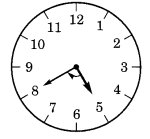(d) Starting from 5 and making 1/2 of a revolution, the clock hand stops at 2.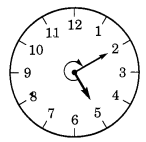Ex 5.2 Class 6 Maths Question 3.
Which direction will you face if you start facingSolution: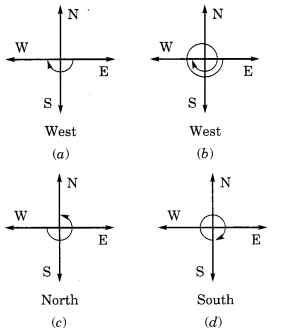Taking one full revolution we will reach back to the original (starting) position. Therefore, it make no difference whether we turn clockwise or anticlockwise.

Ex 5.2 Class 6 Maths Question 4.
What part of a revolution have you turned through if you stand facing
(a) east and turn clockwise to face north?
(b) south and turn clockwise to face east?
(c) west and turn clockwise to face east?
Solution:
(a) If we start from east and reach at north (turning clockwise) 3/4 of a revolution is required.(b) If we start from south turning clockwise to face east, 3/4 of a revolution is required.(c) If we start from west turning clockwise to face east, 1/2 of a revolution is required.Ex 5.2 Class 6 Maths Question 5.
Find the number of right angles turned through by the hour hand of a clock when it goes from
(a)3 to 6
(b) 2 to 8
(c) 5 to 11
(d) 10 to 1
(e) 12 to 9
(f) 12 to 6
Solution:
(a) 3 to 6Starting from 3 to 6, the hour hand turns through 1 right angle.

(b) 2 to 8Starting from 2 to 8, the hour hand turns through 2 right angles.

(c) 5 to 11Starting from 5 to 11, the hour hand turns through 2 right angles.

(d) 10 to 1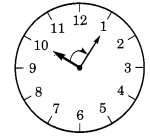Starting from 10 to 1, the hour hand turns through 1 right angle.

(e) 12 to 9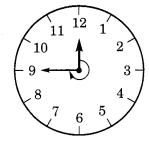Starting from 12 to 9, the hour hand turns through 3 right angles.

(f) 12 to 6Starting from 12 to 6, the hour hand turns through 2 right angles.

Ex 5.2 Class 6 Maths Question 6.
How many right angles do you make if you start facing
(a) south and turn clockwise to west?
(b) north and turn anticlockwise to east?
(c) west and turn to west?
(d) south and turn to north?
Solution:Ex 5.2 Class 6 Maths Question 7.
Where will the hour hand of a clock stop if it starts
(a) from 6 and turns through 1 right angle?
(b) from 8 and turns through 2 right angles?
(c) from 10 and turns through 3 right angles?
(d) from 7 and turns through 2 straight angles?
Solution:
(a) Starting from 6 and turning through 1 right angle, the hour hand stops at 9.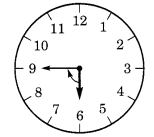(b) Starting from 8 and turning through 2 right angles, the hour hand stops at 2.(c) Starting from 10 and turning through 3 right angles, the hour hand stops at 7.(b) Starting from 7 and turning through 2 right angles, the hour hand stops at 7.### Understanding Elementary Shapes Class 6 Ex 5.3

Ex 5.3 Class 6 Maths Question 1.
Match the following:
(i) Straight angle                  (a) Less than one-fourth of a revolution.
(ii) Right angle                      (b) More than half a revolution.
(iii) Acute angle                    (c) Half of a revolution.
(iv) Obtuse angle                  (d) One-fourth of a revolution.
(v) Reflex angle                     (e) Between 1/4 and 1/2 of a revolution.
–                                                (f) One complete revolution.

Solution:
(i) Straight angle          ↔       (c) Half of a revolution.
(ii) Right angle             ↔       (d) One-fourth of a revolution.
(iii) Acute angle            ↔       (a) Less than one-fourth of a revolution.
(iv) Obtuse angle         ↔        (e) Between 1/4 and 1/2 of a revolution.
(v) Reflex angle            ↔        (f) One complete revolution, right, acute, obtuse or reflex.

Ex 5.3 Class 6 Maths Question 2.
Classify each one of the following anglesSolution:
(a) Acute angle
(b) Obtuse angle
(c) Right angle
(d) Reflex angle
(e) Straight angle
(f) Acute angle

### Understanding Elementary Shapes Class 6 Ex 5.4

Ex 5.4 Class 6 Maths Question 1.
What is the measure of (i) a right angle (ii) a straight angle?
Solution:
(i) Measure of a right angle = 90°
(ii) Measure of a straight angle = 180°

Ex 5.4 Class 6 Maths Question 2.
Say True or False:
(a) The measure of an acute angle < 90°
(b) The measure of an obtuse angle < 90°
(c) The measure of a reflex angle > 180°
(d) The measure of one complete revolution = 360°
(e) If m ∠A = 53° and ∠B = 35°, then m∠A > m∠B.
Solution:
(a) True
(b) False
(c) True
(d) True
(e) True

Ex 5.4 Class 6 Maths Question 3.
Write down the measures of
(a) some acute angles
(b) some obtuse angles
Solution:
(a) 25°, 63° and 72° are acute angles.
(b) 105°, 120° and 135° are obtuse angles.

Ex 5.4 Class 6 Maths Question 4.
Measure the angles given below using the protractor and write down the measure.Solution:
(a) 45°
(b) 125°
(c) 90°
(d) ∠1 = 60°, ∠2 = 90°, ∠3 = 125°

Ex 5.4 Class 6 Maths Question 5.
Which angle has a large measure? First estimate and then measure.
Measure of Angle A =
Measure of Angle B =Solution:
Measure of Angle A = 40°
Measure of Angle B = 60°.

Ex 5.4 Class 6 Maths Question 6.
From these two angles which has large measure? Estimate and then confirm by measuring them.
Solution:
The opening of angle (b) is more than angle (a).∴ Measure of angle (a) = 45°
and the measure of angle (b) = 60°

Ex 5.4 Class 6 Maths Question 7.
Fill in the blanks with acute, obtuse, right or straight:
(a) An angle whose measure is less than that of a right angle is ……… .
(b) An angle whose measure is greater than that of a right angle is ……… .
(c) An angle whose measure is the sum of the measures of two right angles is ……… .
(d) When the sum of the measures of two angles is that of a right angle, then each one of them is ……… .
(e) When the sum of the measures of two angles is that of a straight angle and if one of them is acute then the other should be ……… .
Solution:
(a) acute
(b) obtuse
(c) straight
(d) acute
(e) obtuse

Ex 5.4 Class 6 Maths Question 8.
Find the measure of the angle shown in each figure. (First estimate with your eyes and than find the actual measure with a protractor).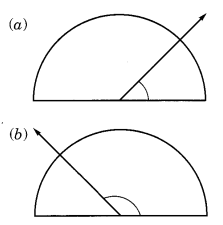Solution:
(a) Measure of the angle = 40°
(b) Measure of the angle = 130°
(c) Measure of the angle = 65°
(d) Measure of the angle = 135°.

Ex 5.4 Class 6 Maths Question 9.
Find the angle measure between the hands of the clock in each figure:Solution:
(i) The angle between hour hand and minute hand of a clock at 9.00 a.m = 90°
(ii) The angle between the hour hand and minute hand of a clock at 1.00 p.m = 30°
(iii) The angle between the hour hand and minute hand of a clock at 6.00 p.m = 180°.

Ex 5.4 Class 6 Maths Question 10.
Investigate: In the given figure, the angle measures 30°. Look at the same figure through a magnifying glass. Does the angle becomes larger? Does the size of the angle change?Solution:
It is an activity. So try it yourself.

Ex 5.4 Class 6 Maths Question 11.
Measure and classify each angle: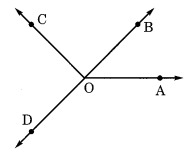Angle Measure Type ∠AOB ∠AOC ∠BOC ∠DOC ∠DOA ∠DOB

Solution:

 Angle Measure Type ∠AOB 40° Acute angle ∠AOC 125° Obtuse angle ∠BOC 85° Acute angle ∠DOC 95° Obtuse angle ∠DOA 140° Obtuse angle ∠DOB 180° Straight angle

### Understanding Elementary Shapes Class 6 Ex 5.5

Ex 5.5 Class 6 Maths Question 1.
Which of the following are models for perpendicular lines:
(a) The adjacent edges of a table top.
(b) The lines of a railway track.
(c) The line segments forming a letter ‘L’.
(d) The letter V.
Solution:
(a) Yes, the adjacent edges of a table top are the models of perpendicular lines.
(b) No, the lines of a railway tracks are parallel to each other. So they are not a model for perpendicular lines.
(c) Yes, the two line segments of‘L’ are the model for perpendicular lines.
(d) No, the two line segments of ‘V’ are not a model for perpendicular lines.

Ex 5.5 Class 6 Maths Question 2.∴ ∠PAY = 90°Ex 5.5 Class 6 Maths Question 3.
There are two set-squares in your box. What are the measures of the angles that are formed at their corners? Do they have any angle measure that is common?
Solution:
The figures of the two set-squares are given below: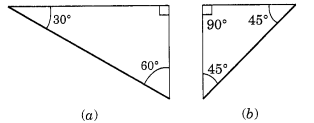The measure angles of triangle (a) are : 30°, 60° and 90°.
The measure angles of triangle (b) are 45°, 45° and 90°.
Yes, they have a common angle of measure 90°.

Ex 5.5 Class 6 Maths Question 4.
Study the diagram. The line l is perpendicular to line m.
(a) Is CE = EG?
(b) Does PE bisects CG?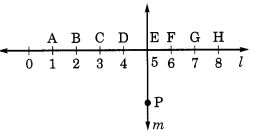(c) Identify any two line segments for which PE is the perpendicular bisector.
(d) Are these true?
(i) AC > FG
(ii) CD = GH
(iii) BC < EH
Solution:
(a) Yes,
Since, CE = 2 units and EG = 2 units
Hence, CE = EG.
(b) Yes, PE bisects CG(d) (i) True (ii) True (iii) True

### Understanding Elementary Shapes Class 6 Ex 5.6

Ex 5.6 Class 6 Maths Question 1.
Name the types of following triangles:
(а) Triangle with lengths of sides 7 cm, 8 cm and 9 cm.
(b) ∆ABC with AB = 8.7 cm, AC = 7 cm and BC = 6 cm.
(c) ∆PQR such that PQ = QR = PR = 5 cm.
(d) ∆DEF with m∠D = 90°
(e) ∆XYZ with m∠Y = 90° and XY = YZ.
(f) ∆LMN with m∠L = 30° m∠M = 70° and m∠N = 80°.
Solution:
(a) Lengths of the sides of a triangle are given as: 7 cm, 8 cm and 9 cm.
Since, all sides of the given triangle are different.
Hence, it is a Scalene triangle.
(b) Given that: AB = 8.7 cm, AC = 7 cm and BC = 6 cm
Here AB ≠ AC ≠ BC Hence, ∆ABC is Scalene triangle.
(c) Given that: PQ = QR = PR = 5 cm
Since all sides are equal.
Hence, it is an equilateral triangle.
(d) Given that: In ∆DEF, m∠D = 90°
Hence it is a right angled triangle.
(e) Given that: In ∆XYZ, m∠Y = 90° and XY = YZ
Hence it is a right angled triangle.
(f) Given that: ∆LMN, m∠L = 30°, m ∠M = 70° and m∠N = 80°.
Hence it is an acute angled triangle.

Ex 5.6 Class 6 Maths Question 2.
Match the following:
Measure of triangle                                                     Type of triangle
(i) 3 sides of equal length                                                    (a) Scalene
(ii) 2 sides of equal length                                                  (b) Isosceles right angled
(iii) All sides are of different length                                  (c) Obtuse angled
(iv) 3 acute angles                                                                (d) Right angled
(v) 1 right angle                                                                     (e) Equilateral
(vi) 1 obtuse angle                                                                 (f) Acute angled
(vii) 1 right angle with two sides of equal length            (g) Isosceles
Solution:
(i) ↔ (e)
(ii) ↔ (g)
(iii) ↔ (a)
(iv) ↔ (f)
(v) ↔ (d)
(vi) ↔ (c)
(vii) ↔ (b)

Ex 5.6 Class 6 Maths Question 3.
Name each of the following triangles in two different ways: (You may judge the nature of the angle by observation)Solution:
(a) (i) Acute angled triangle
(ii) Isosceles triangle

(b) (i) Right angled triangle
(ii) Scalene triangle

(c) (i) Obtuse angled triangle
(ii) Isosceles triangle

(d) (i) Right angled triangle
(ii) Isosceles triangle

(e) (i) Acute angled triangle
(ii) Equilateral triangle

(f) (i) Obtuse angled triangle
(ii) Scalene triangle.

Ex 5.6 Class 6 Maths Question 4.
Try to construct triangles using matchsticks. Some are shown here. Can you make a triangle with
(a) 3 matchsticks?
(b) 4 matchsticks?
(c) 5 matchsticks?
(d) 6 matchsticks?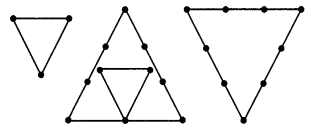(Remember you have to use all the available matchsticks in each case)
Name the type of triangle in each case.
If you cannot make a triangle, give of reasons for it.
Solution:
(a) Yes, we can make an equilateral triangle with 3 matchsticks.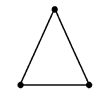(b) No, we cannot make a triangle with 4 matchsticks.
(c) Yes, we can make an isosceles triangle with five matchsticks.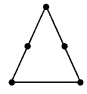(d) Yes, we can make an equilateral triangle with 6 matchsticks.### Understanding Elementary Shapes Class 6 Ex 5.7

Ex 5.7 Class 6 Maths Question 1.
Say True or False:
(a) Each angle of a rectangle is a right angle.
(b) The opposite sides of a rectangle are equal in length.
(c) The diagonals of a square are perpendicular to one another.
(d) All the sides of a rhombus are of equal length.
(e) All the sides of a parallelogram are of equal length.
(f) The opposite sides of a trapezium are parallel.
Solution:
(a) True
(b) True
(c) True
(d) True
(e) False
(f) False

Ex 5.7 Class 6 Maths Question 2.
Give reasons for the following:
(a) A square can be thought of as a special rectangle.
(b) A rectangle can be thought of as a special parallelogram.
(c) A square can be thought of as a special rhombus.
(d) Square, rectangles, parallelograms are all quadrilaterals.
(e) Square is also a parallelogram.
Solution:
(a) A square has all the properties as that of rectangle. So, it is a special rectangle.
(b) A rectangle has the same properties as that of parallelogram. So, it is a special parallelogram.
(c) A square has the same properties as that of a rhombus. So, it is a special rhombus.
(d) Square, rectangles and parallelogram are all quadrilateral as they are all enclosed by four sides.

Ex 5.7 Class 6 Maths Question 3.
A figure is said to be regular if its sides are equal in length and angles are equal in measure. Can you identify the regular quadrilateral?
Solution:
Square is only the regular quadrilateral with equal sides and equal angles.
Therefore, square is a regular quadrilateral.

Understanding Elementary Shapes Class 6 Ex 5.8

Ex 5.8 Class 6 Maths Question 1.
Examine whether the following are polygons. If any one among them is not, say why?Solution:
(a) The given figure is not closed. Therefore, it is not a polygon.
(b) The given figure is a polygon.
(c) The given figure is not a polygon because every polygon is enclosed with line segments.
(d) The given figure is not a polygon because it is enclosed by an arc and two line segments.

Ex 5.8 Class 6 Maths Question 2.
Name the polygon.Make two more examples of each of these.
Solution:(b) A Triangle
Examples:(c) A Pantagon
Examples:(d) A Octagon
Examples: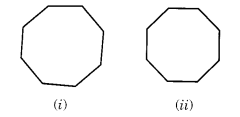Ex 5.8 Class 6 Maths Question 3.
Draw a rough sketch of a regular hexagon. Connecting any three of its vertices, draw a triangle. Identify the type of the triangle you have drawn.
Solution:
ABCDEF is a rough sketch of a regular hexagon. If we join any three vertices like D, A and B, we get a scalene triangle DAB.But if we join the alternate vertices, we get an equilateral triangle EAC.

Ex 5.8 Class 6 Maths Question 4.
Draw a rough sketch of a regular octagon. (Using squared paper if you wish). Draw a rectangle by joining exactly four of the vertices of the octagon.
Solution:
ABCDEFGH is a rough sketch of regular octagon. GHCD is the rectangle formed by joining the four vertices of the given octagon.Ex 5.8 Class 6 Maths Question 5.
A diagonal is a line segment that joins any two vertices of the polygon and is not a side of the polygon. Draw a rough sketch of a pentagon and draw its diagonals.
Solution:
A B C D E is the rough sketch of a pentagon.
By joining its any two vertices, we get, the following diagonals.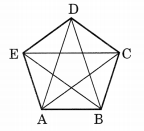### Understanding Elementary Shapes Class 6 Ex 5.9

Ex 5.9 Class 6 Maths Question 1.
Match the following:Give two examples of each shape.
Solution:
(a) 4 ↔ (ii)
Examples:
(i) An ice-cream cone
(ii) Birthday cap

(b) ↔ (iv)
Examples:
(i) Tennis ball
(ii) Cricket ball

(c) ↔ (v)
Examples:
(ii) A lawn roller

(d) ↔ (iii)
Examples:
(i) Math book
(ii) A brick

(e) ↔ (i)
Examples:
(i) A diamond
(ii) Egypt-Pyramids

Ex 5.9 Class 6 Maths Question 2.
What shape is
(b) A brick?
(b) A matchbox?
Solution:
(a) Shape of instrument box is cuboid.
(b) Shape of a brick is cuboid.
(c) Shape of a matchbox is cuboid.
(d) Shape of a road-roller is cylinder.
(e) Shape of a sweet laddu is sphere.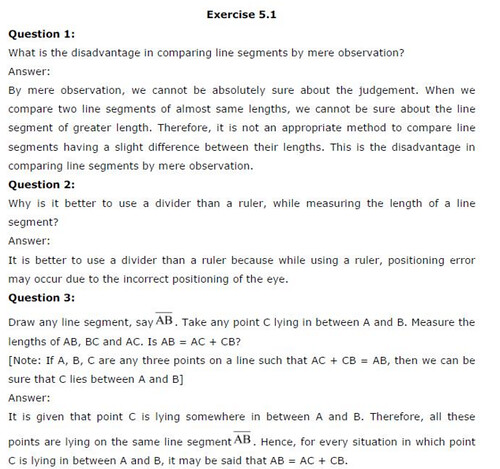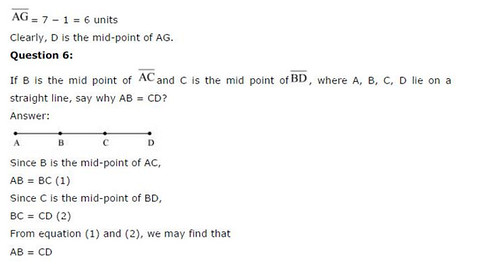## SabDekho

The Complete Educational Website